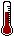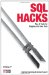# Hack 22. Generate Quarterly ReportsA quarterly report aggregates three months' worth of figures. SQL has all the functions you need to get this aggregation.

Suppose you have figures that you need to report on by quarter. The source of your data is just a list of dates and values, as shown in Table 4-4.

Table 4-4. The sale table

whn amount
2005-01-06 2
2005-03-14 8
2005-04-02 4

In a quarterly report, you need to SUM all the figures relating to January, February, and March into Q1. So the first two rows of Table 4-4 contribute to the Q1 total for 2005. The 2005-04-02 row occurred in April, so you should add it to the Q2 total for 2005.

You can use the MONTH function to extract the month as a number, with January, February, and March appearing as 1, 2, and 3.

If you also group on the year, each quarter of your input will correspond to exactly one cell in the output grid:

```mysql> SELECT YEAR(whn) AS yr
-> ,SUM(CASE WHEN MONTH(whn) IN (1,2,3) THEN amount END) AS Q1
-> ,SUM(CASE WHEN MONTH(whn) IN (4,5,6) THEN amount END) AS Q2
-> ,SUM(CASE WHEN MONTH(whn) IN (7,8,9) THEN amount END) AS Q3
-> ,SUM(CASE WHEN MONTH(whn) IN (10,11,12) THEN amount END) AS Q4
-> FROM sale
-> GROUP BY YEAR(whn);
+------+------+------+------+------+
| yr | Q1 | Q2 | Q3 | Q4 |
+------+------+------+------+------+
| 2005 | 10 | 40 | 80 | 660 |
| 2006 | 30 | 20 | NULL | NULL |
+------+------+------+------+------+```

Unfortunately, the YEAR and MONTH functions are not implemented in Oracle. However, the SQL standard EXTRACT function works just as well:

```SQL> SELECT EXTRACT(YEAR FROM whn) AS yr
2 ,SUM(CASE WHEN EXTRACT(MONTH FROM whn) IN (1,2,3)
3 THEN amount END) AS Q1
4 ,SUM(CASE WHEN EXTRACT(MONTH FROM whn) IN (4,5,6)
5 THEN amount END) AS Q2
6 ,SUM(CASE WHEN EXTRACT(MONTH FROM whn) IN (7,8,9)
7 THEN amount END) AS Q3
8 ,SUM(CASE WHEN EXTRACT(MONTH FROM whn) IN (10,11,12)
9 THEN amount END) AS Q4
10 FROM sale
11 GROUP BY EXTRACT(YEAR FROM whn);

YR Q1 Q2 Q3 Q4
---------- ---------- ---------- ---------- ----------
2005 10 40 80 660
2006 30 20```

4.4.1. Hacking the Hack

You may want to pivot the rows and columns of the report. In standard SQL, you have to apply some math:

```mysql> SELECT FLOOR((EXTRACT(MONTH FROM whn)-1)/3)+1 AS Quarter
-> ,SUM(CASE WHEN EXTRACT(YEAR,whn)=2005 THEN amount END) AS Y2005
-> ,SUM(CASE WHEN EXTRACT(YEAR,whn)=2006 THEN amount END) AS Y2006
-> FROM sale
-> GROUP BY FLOOR((EXTRACT(MONTH FROM whn)-1)/3)+1;
+---------+-------+-------+
| Quarter | Y2005 | Y2006 |
+---------+-------+-------+
| 1 | 10 | 30 |
| 2 | 40 | 20 |
| 3 | 80 | NULL |
| 4 | 660 | NULL |
+---------+-------+-------+```

The expression FLOOR((MONTH(whn)-1)/3)+1 calculates the quarter for the input date whn. You can see how it works if you look at the calculation one step at a time:

```mysql> SELECT whn, EXTRACT(MONTH FROM whn) 'Month',
-> EXTRACT(MONTH FROM whn)-1 'Subtract 1',
-> (EXTRACT(MONTH FROM whn)-1)/3 'Divide by 3',
-> FLOOR((EXTRACT(MONTH FROM whn)-1)/3) 'Ignore Fraction',
-> FLOOR((EXTRACT(MONTH FROM whn)-1)/3)+1 'Add 1'
-> FROM sale
-> WHERE YEAR(whn)=2006;
+------------+-------+------------+-------------+-----------------+-------+
| whn | Month | Subtract 1 | Divide by 3 | Ignore Fraction | Add 1 |
+------------+-------+------------+-------------+-----------------+-------+
| 2006-01-01 | 1 | 0 | 0.0000 | 0 | 1 |
| 2006-02-01 | 2 | 1 | 0.3333 | 0 | 1 |
| 2006-03-01 | 3 | 2 | 0.6667 | 0 | 1 |
| 2006-04-01 | 4 | 3 | 1.0000 | 1 | 2 |
| 2006-05-01 | 5 | 4 | 1.3333 | 1 | 2 |
+------------+-------+------------+-------------+-----------------+-------+```

Each vendor has a function to extract the QUARTER and the YEAR from a date. In MySQL, these functions are QUARTER and YEAR:

```mysql> SELECT QUARTER(whn)
-> ,SUM(CASE WHEN YEAR(whn)=2005 THEN amount END) AS Y2005
-> ,SUM(CASE WHEN YEAR(whn)=2006 THEN amount END) AS Y2006
-> FROM sale
-> GROUP BY QUARTER(whn);
+--------------+-------+-------+
| QUARTER(whn) | Y2005 | Y2006 |
+--------------+-------+-------+
| 1 | 10 | 30 |
| 2 | 40 | 20 |
| 3 | 80 | NULL |
| 4 | 660 | NULL |
+--------------+-------+-------+```

The trick is to GROUP BY the quarter and use the CASE statement to extract only one year in each column. There are some database-specific variations to keep in mind:

• MySQL uses QUARTER(whn) and YEAR(whn) as shown.
• In SQL Server, you can use DATEPART(QUARTER,whn) and YEAR(whn).
• In Oracle, you can use TO_CHAR(whn,'Q') and TO_CHAR(whn,'YYYY') for the quarter and year.
• In PostgreSQL, you can use EXTRACT(QUARTER FROM whn) and EXTRACT(YEAR FROM whn).
• In Access, you can use DatePart("q", whn) and YEAR(whn).SQL Hacks
ISBN: 0596527993
EAN: 2147483647
Year: 2004
Pages: 147